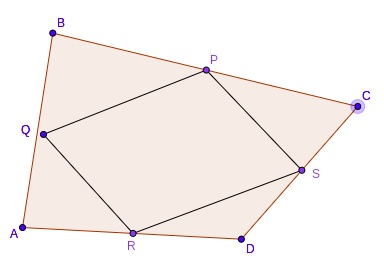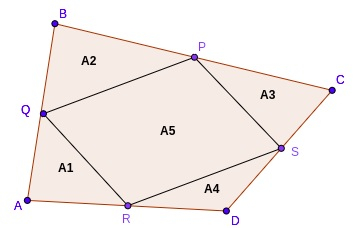# C++ Perimeter and Area of Varignon’s Parallelogram

C++Server Side ProgrammingProgramming

Varignon’s Parallelogram is formed by joining midpoints of each side of a quadrilateral. Let’s say we have a quadrilateral ABCD. The midpoints of each side are P, Q, R, and S. If we connect all the midpoints, it will always form a parallelogram PQRS known as Varignon’s Parallelogram.In this tutorial, we will discuss how to find the perimeter and area of Varignon’s Parallelogram with the given two diagonals and the area of a quadrilateral, for example −

Input: d1 = 6, d2 = 9, Area = 12
Output:
Perimeter = 15
Area = 6

Input: d1 = 11, d2 = 13, Area = 32
Output:
Perimeter = 24
Area = 16

## Approach to Find the Solution

In triangle P and Q are midpoints of AB, AC respectively,

By midpoint theorem, PQ = (1/2)*AC

Similarly applying theorem to triangle ADC, RS = (1/2)*BD,

So PQ=RS=(1/2)*AC and PS=QR=(1/2)*BD

The perimeter of PQRS = AC + BD(sum of diagonals)

EF=GH=(1/2)*AC and EH=FG=(1/2)*BDFor the Area of PQRS, we divide the figure into four triangles, and areas of four triangles are,

similarly, A2=(1/4)*area of ABC

A3=(1/4)*area of BCD

A4=(1/4)*area of ACD.

A1 + A2 + A3 + A4 = (1/4)*(area of triangles ACD+ABC+BCD+BAD)

= (¼) * 2* area of ABCD

= (½) * area of quad ABCD

Now A1 + A2 + A3 + A4 = (½) * area of quad ABCD

It means A5 = (½) * area of quad ABCD

So Area of parallelogram PQRS = (½) * area of quad ABCD

Now we can find the perimeter and area of PQRS by just applying the formula using C++.

## Example

C++ Code for the Above Approach

#include <bits/stdc++.h>
using namespace std;
int main(){
float d1 = 6, d2 = 9, area_ABCD = 12;
float area_PQRS = area_ABCD/2;
float perimeter = d1 + d2;
cout << "Area of parallelogram PQRS = " << area_PQRS << " and perimeter = " << perimeter;
return 0;
}


## Output

Area of parallelogram PQRS = 6 and perimeter = 15

## Conclusion

In this tutorial, we discussed Varignon's Parallelogram and how to find the area and perimeter. We discussed the derivation of perimeter and area of a parallelogram using the midpoint theorem. We also discussed the C++ program for this problem which we can do with programming languages like C, Java, Python, etc. We hope you find this tutorial helpful.